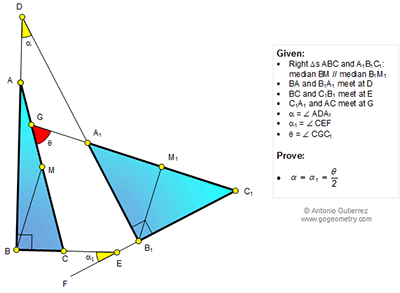## Monday, July 6, 2015

### Geometry Problem 1128: Right Triangles, Medians, Parallel, Angles, Congruence, Half the measure

Geometry Problem. Post your solution in the comments box below.
Level: Mathematics Education, High School, Honors Geometry, College.

Click the diagram below to enlarge it.#### 1 comment:

1.http://s7.postimg.org/ccpzrcdez/pro_1128.png

Observe that quadrilateral DBEB1 is cyclic so α = α 1
Let B1M1 cut BE extension at N
Let b=∠ (MBC) => ∠ (MCB)= β
Since BM//B1M1 => ∠ (M1Nx)= β
And ∠ (CFE)= 180- β - α
And ∠ (M1B1C1)= β - α =∠ (M1C1B1)
In triangle GFC1 θ = 180-(180- β - α)-( β - α)= 2. α